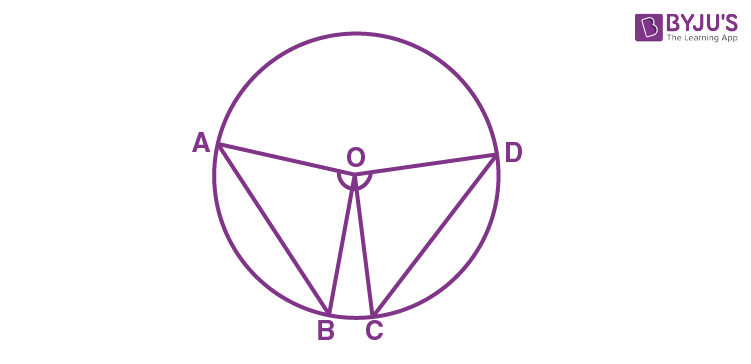# Angle Subtended by a Chord at a Point

In Mathematics, a circle is a two-dimensional geometrical figure, where all the points on the surface of a circle are equidistant from the center point called the radius. The chord of a circle is the line segment that joins two points on the circumference of a circle. If you draw two or more equal chords of a circle and determine the angles subtended by them at the center, you will observe that the angles subtended by them at the center are equal. In this article, we will learn the theorems related to the angle subtended by a chord at a point in detail.

## Angle Subtended by a Chord at a Point – Theorem

Theorem: Equal chords of a circle subtend equal angles at the center.

### Proof

Consider a circle and draw two equal chords AB and CD of a circle with center “O” as shown in the figure.To prove: ∠ AOB = ∠ COD.

From the triangles, AOB and COD, we get

OA = OC (Radii of a circle)

OB = OD (Radii of a circle)

AB = CD (Equal chords)

By, using Side-Side-Side (SSS Rule), we can write:

∆ AOB ≅ ∆ COD

As the triangles are congruent, the angles should be of equal measurement.

Therefore, ∠ AOB = ∠ COD [Using Corresponding parts of the congruent triangle (CPCT)]

Hence, the theorem “Equal chords of a circle subtend equal angles at the center” is proved.

## Converse Theorem

If the angles subtended by the chords of a circle at the center are equal, then the chords are equal.

In the converse theorem, if ∠AOB is equal to ∠COD, we need to prove that the chord AB = CD.

This can be again proved with the help of CPCT and SSS rules.

To learn more Maths theorems and all Maths-related concepts, register with BYJU’S – The Learning App and understand the concepts easily by exploring more videos.